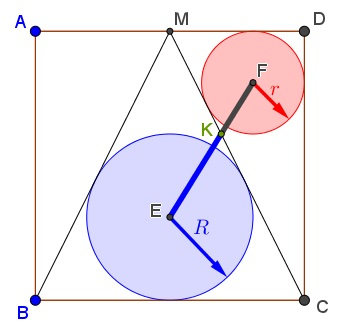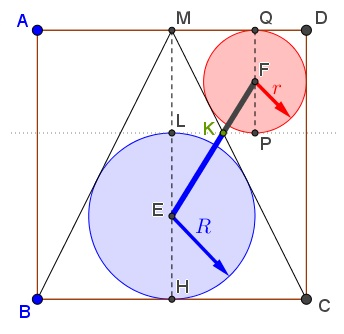# Golden Ratio in Square

### Problem

The following long overdue observation was proposed by Ercole Suppa (Italy) at the Peru Geometrico facebook group.

$M$ is the midpoint of side $AD$ of square $ABCD.$ Let $R$ be the inradius of $\Delta BCM,$ $r$ the inradius of $\Delta CDM.$Then $\displaystyle \frac{R}{r}=\frac{\sqrt{5}+1}{2}=\varphi,$ the Golden Ratio.

### Proof 1

Let $AB=2.$ Then $BM=CM=\sqrt{5}.$ We'll use a formula for the area of a triangle: the semiperimeter times the inradius. Thus, since $[\Delta BCM]=2[\Delta CDM],$

$(2\sqrt{5}+2)\cdot R=2\cdot (3+\sqrt{5})\cdot r,$

from which

$\displaystyle \frac{R}{r}=\frac{\sqrt{5}+3}{\sqrt{5}+1}=\frac{(\sqrt{5}+3)(\sqrt{5}-1)}{4}=\frac{\sqrt{5}+1}{2}=\varphi.$

### Proof 2

WLOG, let $AD=2$. Let, $\angle MBC=\angle DMC=\theta$.

\displaystyle \begin{align} &\tan \theta=\frac{2\tan \frac{\theta}{2}}{1-\tan^2 \frac{\theta}{2}}=\frac{DC}{DM}=2 \\ &\tan\frac{\theta}{2}=\frac{\sqrt{5}-1}{2}=\varphi-1.~\text{(Dropping the negative root)} \end{align}

\displaystyle \begin{align} \tan \angle DMF&=\tan~\frac{\theta}{2}=\frac{r}{MD-r}\\ &\Rightarrow \varphi-1=\frac{r}{1-r}\\ &\Rightarrow r=\frac{\varphi-1}{\varphi}. \end{align}

\displaystyle \begin{align} \tan~\angle EBC&=\tan~\frac{\theta}{2}=\frac{R}{1}\\ &\Rightarrow R=\varphi-1. \end{align}

Thus,

$\displaystyle \frac{R}{r}=\varphi.$

### Extra

We shall prove a little more, viz.,

Let $E$ and $F$ be the incenters of triangles $BCM$ and $CDM$ and $K$ the intersection of $EF$ and $CM.$Then $\displaystyle \frac{EK}{FK}=\varphi,$ the Golden Ratio.

### Proof of Extra

Let $L$ be the topmost point of $(E),$ $H$ its point of tangency with $BC$ (the midpoint of $BC),$ $P$ and $Q$ the lowest and the topmost points of $(F).$Then, $L,K,P$ are collinear and $LP\parallel BC:$

\displaystyle\begin{align}R&=\frac{2}{2(\sqrt{5}+1)}=\frac{\sqrt{5}-1}{4},\\ r&=\frac{1}{\sqrt{5}+3}=\frac{3-\sqrt{5}}{4},\\ ML&=2-2\cdot\frac{\sqrt{5}-1}{4}=\frac{3-\sqrt{5}}{2},\\ PQ&=2\cdot \frac{3-\sqrt{5}}{4}=\frac{3-\sqrt{5}}{2}. \end{align}

This makes triangles $EKL$ and $FKP$ similar, so that $\displaystyle \frac{EK}{FK}=\frac{EH}{FP}=\frac{R}{r}=\varphi.$

### Extra 2

Note that, from the proof above, the triangles $HKL$ and $QKP$ are also similar, so that $\displaystyle \frac{HK}{QK}=\varphi:$And if so, triangles $MKQ$ and $CKH$ are similar, implying $\displaystyle \frac{CK}{MK}=\varphi.$

### Golden Ratio